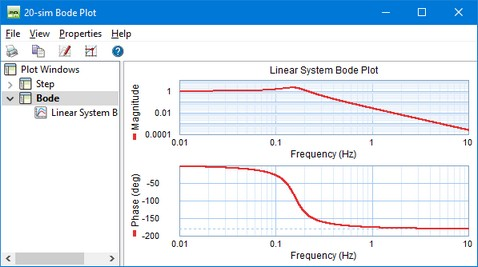﻿ 20-sim webhelp > Toolboxes > Control Toolbox > Controller Design Editor > Bode Plots

# Bode Plots

Bode Plots show the amplitude and phase of a linear system as function of the frequency. Bode plots can be shown for every 20-sim model through Linearization. During Linearization you are asked to enter the input variable and output variable for which linearization should be performed. After that the linear system is calculated and shown in the Linear System Editor. From the Linear System Editor you can generate a bode plot. These actions can also be predefined using the Frequency Response command of the Properties menu.20-sim automatically generates a range of logarithmically displayed frequencies, based on the system dynamics. With the Plot Properties command (right mouse menu), you can change this horizon and recalculate the bode response (click the Bode command again).

The magnitude part of the plot can be displayed in dB or in absolute values. The phase part can be displayed in radians or degrees. The frequency can be displayed in radians per second or in Hz.

## Plot Options

Using the toolbar or the right mouse menu, you can use various options:

 • Plot Properties: Set the plot properties.
 • Numerical Values: Inspect numerical values.
 • Magnitude (dB): Display magnitude in decibels
 • Magnitude (-): Display magnitude in absolute values.